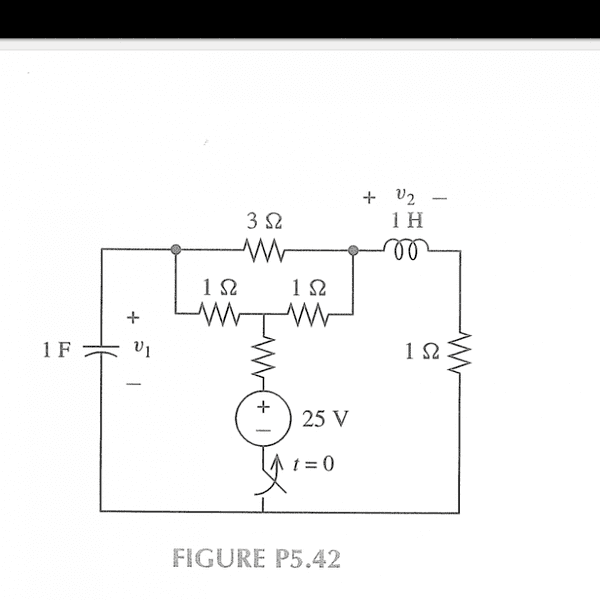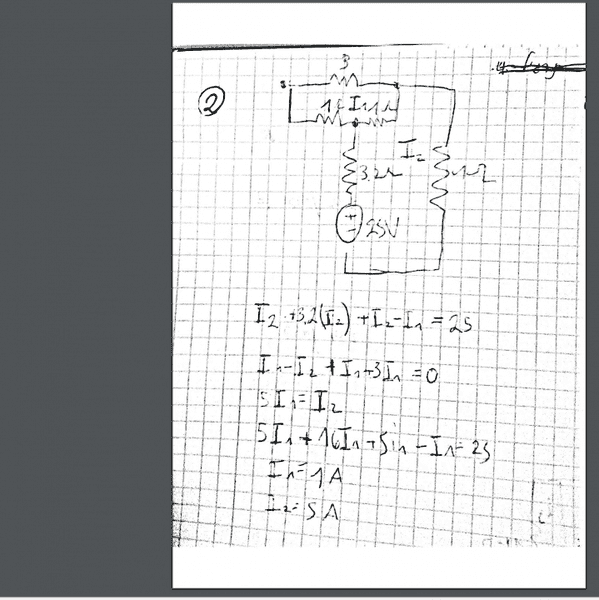• sdfanm16

#### sdfanm16## Homework Statement

{Moderator edit: The unlabeled resistance is given to be 3.2 Ω }

finding v1 and v2 at t=0+ and t=0- is needed

## The Attempt at a Solutionok I've found the currents by mesh analysis but how can i get the voltage?What voltage is in the inductor or capacitor if its a short/open circuit?
Tal

Now you know the current flowing, you can work out the voltage at any point or the voltage across any component. Find the voltage across the capacitor.

Say find the voltage at the RH end of the 3Ω.
Then the junction of the 1Ω Rs
Then at the 1Ω 3Ω junction. Voila.

finding v1 and v2 at t=0+ and t=0- is needed
The subject says: "dc steady state", yet t=0+ is far from steady state. So which are you wanting to find?

You seem to know that the unlabelled resistor is 3.2 Ω?

The subject says: "dc steady state", yet t=0+ is far from steady state. So which are you wanting to find?

You seem to know that the unlabelled resistor is 3.2 Ω?
yes I've forgot to wrote this. it's given.

My guess was that you had to find v1 at t=0- when it is stable and to find v2 at t=0+ when it is maximum.
v2 at t=0- is trivial and v1 at t =0+ I would assume is the same as at t=0- , otherwise you would need to know how + t is!
As t →0 both become more accurate.